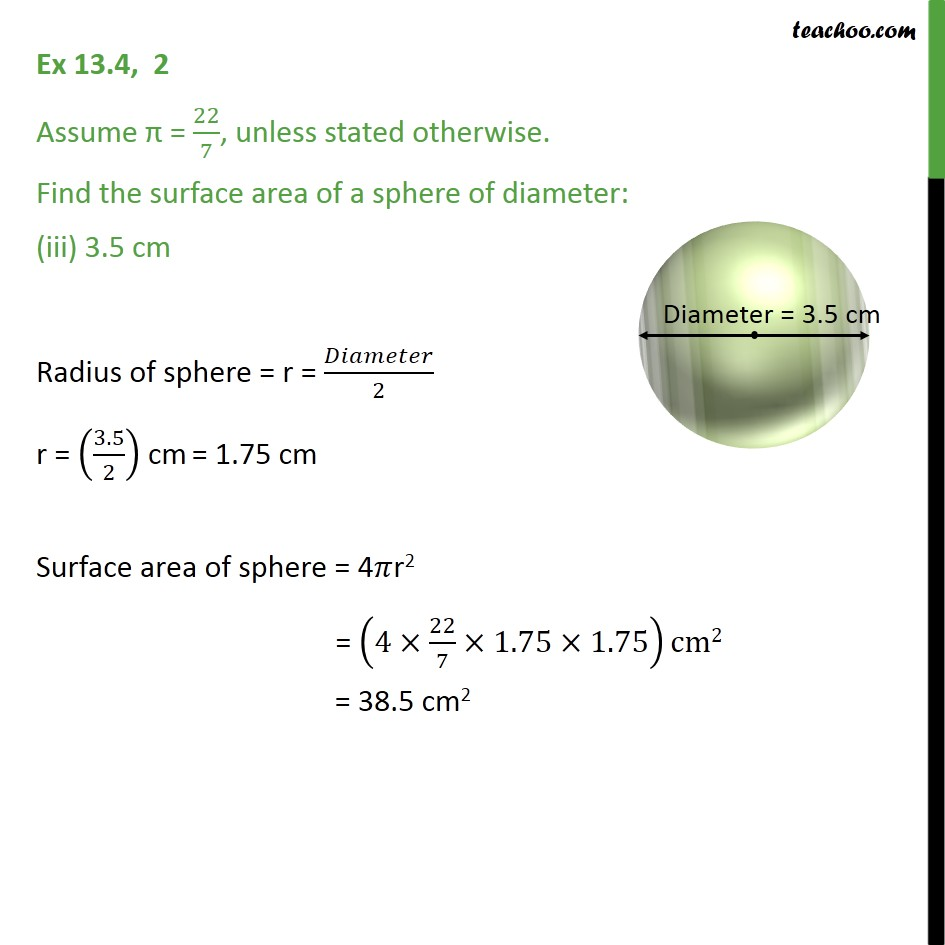1. Chapter 13 Class 9 Surface Areas and Volumes (Term 2)
2. Serial order wise
3. Ex 13.4

Transcript

Ex 13.4, 2 Assume = 22/7, unless stated otherwise. Find the surface area of a sphere of diameter: (iii) 3.5 cm Radius of sphere = r = /2 r = (3.5/2) cm = 1.75 cm Surface area of sphere = 4 r2 = (4 22/7 1.75 1.75)cm2 = 38.5 cm2